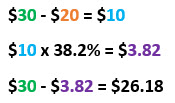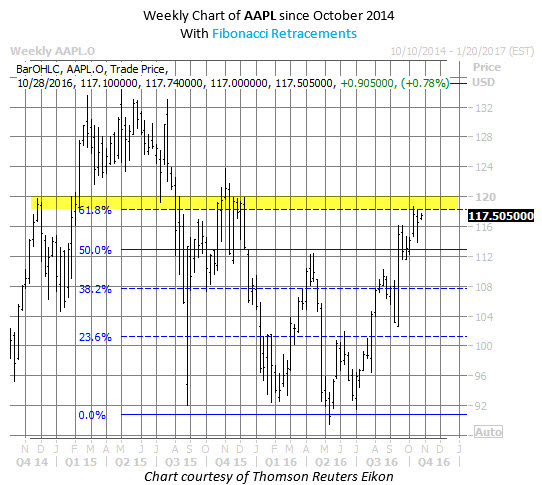# Using Fibonacci Retracement Levels to Define Price Trends

## levels are often useful in defining short- and long-term price trends for a stock or sector

Oct 27, 2016 at 2:51 PM

Technical analysis is an important aspect of stock and option trading methodology. In conjunction with fundamental and sentimental analysis -- what we at Schaeffer's refer to as the three-pronged Expectational Analysis approach -- traders can gain possible insight into a stock's trends and future behavior. Percentage-retracement levels, and the Fibonacci sequence in particular, are often useful in defining short- and long-term price trends in a security or sector.

Fibonacci numbers follow an integer sequence where every number is the sum of the two proceeding numbers, and where each number in the sequence is approximately 1.618 times greater than the number before it:

0, 1, 1, 2, 3, 5, 8, 13, 21, 34, 55, 89, 144…

While the significance of Fibonacci numbers is vast, and stretches from computer programming to the many occurrences found in the natural world, and everything in between, Fibonacci retracement levels can also give stock traders another technical tool to gain valuable insight into an equity's price trend behavior by acting as a level of support or resistance.

Finding a Fibonacci retracement level involves taking Fibonacci number and dividing it by another number that appears later in the chain. Dividing one number by another number immediately to its right gives you the approximate retracement ratio of 61.8%, or the "golden ratio," such as 55 divided by 89. Another key retracement level can be found by dividing one number by another number two places to its right in the Fibonacci chain, such as 55 divided by 144, which is approximately 38.2%.

Key Fibonacci retracement levels include:

• 23.6% (A number divided by another number three places to the right)
• 38.2% (A number divided by another number two places to the right)
• 50% (1 divided by 2)
• 61.8% (A number divided by another number one place to the right)
• 76.4% (100% – 23.6%)
• 100% (1 divided by 1)

Finding a specific stock's Fibonacci retracement level involves subtracting a high point from a low point for an underlying equity to find the retracement levels. For example, if stock XYZ peaked at \$30 in April, and bottomed out at \$20 during a subsequent pullback, XYZ's Fibonacci retracement levels would be based on the difference of \$10. So, to find XYZ's 38.2% retracement level, a trader would:This information alerts traders to a potential floor or ceiling at or near that price point. This phenomenon can be seen in recent Apple Inc. (NASDAQ:AAPL) price action. Prior to the company's earnings release this week, the stock was facing resistance in the \$118-\$120 region, which contained AAPL's rally attempt in late 2015. This area also represents a 61.8% Fibonacci retracement -- that "golden ratio" -- of the stock's plunge from mid-2015 to May 2016.If you are not making money with options, you aren’t buying options like this…

There is no options strategy that more perfectly approaches trading the fastest moving and most volatile stocks available in the marketplace than this one. In fact, there is no strategy that better utilizes put options for optimal returns and a real trading edge over other traders in the exact same market. New options traders fail out at an incredible rate without proper trade research, execution timing, and option picking. Capitalize on Schaeffer’s 100+ years of options trading excellence with the most coveted product launch in company history. Don't waste another second... join us right now before the next round of trades are released!